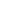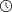Back to articles list- 3 minutes read

# Theory and History: Why are Relational Databases “Relational”?

Many people wonder why relational databases are called “relational.” Some think that it’s because of a logical entity-relationship model you often start your design with. Or, because you have tables and relationships (aka foreign keys) between them. But that’s not the case.

The name comes from the mathematical notion of “relation.” It all started with E. F. Codd who in 1970 (in the article A Relational Model of Data for Large Shared Data Banks) proposed something now called relational algebra as the mathematical foundation of databases.

In mathematics, a relation is a subset of a Cartesian product. A Cartesian product A × B is the set of all pairs (a,b) where a ∈ A, b ∈ B; a product A × B × C is the set of all triples (a,b,c) where a ∈ A, b ∈ B, c ∈ C; and so on. So, a relation between three sets is a certain set of triples. For example, a relation between the sets {0,1,2}, {0,1,2} and {-2,-1,0,1,2} may be defined like this:

```r = { (0,0,0), (0,1,-1), (0,2,-2),
(1,0,1), (1,1,0),  (1,2,-1),
(2,0,2), (2,1,1),  (2,2,0) }
```

If you’re used to thinking that a relation is something like “a divides b” or “a is less than b” then this approach might be difficult to understand. But the difference is really in your head . Instead of giving explanation of a relation you enumerate the facts that hold. The relation r above is subtraction in {0,1,2}: (x,y,z) ∈ r if x-y = z. An infinite enumeration

```{ (0,1), (0,2), (1,2), (0,3), (1,3), (2,3), (0,4), ... }
```

is the definition of the relation “a is less than b.”

### What does this have to do with databases?

You’ll see the connection, if I write the relation r like this:

```----------
|    r   |
|--------|
| 0| 0| 0|
| 0| 1|-1|
| 0| 2|-2|
| 1| 0| 1|
| 1| 1| 0|
| 1| 2|-1|
| 2| 0| 2|
| 2| 1| 1|
| 2| 2| 0|
----------
```

A table in a database is a relation – an enumeration of the facts that hold. A typical example of a table, this one taken from the original Codd’s paper:

```----------------------------------------
|                supply                |
|--------------------------------------|
| supplier | part | project | quantity |
|--------------------------------------|
|     1    |   2  |    5    |    17    |
|     1    |   3  |    5    |    23    |
|     2    |   3  |    7    |     9    |
|     2    |   7  |    5    |     4    |
|     4    |   1  |    1    |    12    |
----------------------------------------
```

Anything you do with tables in a database yields a relation. The result of an SQL query is a relation.

```select supplier, part from supply where quantity > 10;
-------------------
| supplier | part |
|-----------------|
|     1    |   2  |
|     1    |   3  |
|     4    |   1  |
-------------------
```

view (stored SQL query) is a relation, too.

Everything in a database is a relation and thus databases are “relational.”

Design your own relational database with Vertabelo.

1. The big word for the explanation approach is “intensional,” for the enumeration approach it’s “extensional.” As the names suggest, they are two alternative, dual approaches to defining and handling mathematical objects.